• Call Now

1800-102-2727•

# Atomic Number, Mass number: Definitions, examples and practice problems

Aadhar card, PAN number, Passport number, etc represent the unique identity of a person. In the same way, what according to you is the most unique identity of an element?

The concept of atomic mass dominated science for a long time, we have seen many attempts to develop the periodic table based on atomic mass but after the discovery of x-rays, electrons, and nuclei in an experiment Henry Mosley bombarded different metals with a beam of electrons. The produced spectrum of x-ray of each element depends on the position of the list of hydrogen to uranium. He discovered a relation between wavelength and an atomic number of elements. He predicted a few missing elements with their atomic numbers. So, the atomic number, not atomic mass decides what the element was.

## General representation of an elementAtomic number

The atomic number of an element is the number of protons present in the nucleus. An atomic number is used to differentiate one element from another.

Eg. C612; carbon has 6 protons in its nucleus and its atomic number is 6.

O816; oxygen has 8 protons in its nucleus and its atomic number is 8.

## Discovery of atomic number

Henry Mosley bombarded different metals with a beam of electrons. The frequency of the produced x-ray from the different elements was plotted with their corresponding atomic number. A straight line is obtained between the square root of the frequency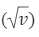of produced x-ray and atomic number(Z).

Mathematical form, v=a(Z-b)

Where, v= frequency of emitted x - ray

Z= atomic number of element

a & b are constants.## Mass numberThe mass number of an element is the number of nucleons (proton and neutron) present in the nucleus.

The number of protons = atomic number (Z)

Number of neutron (Z) = mass number - atomic number

Eg. C612; carbon has 6 proton and 6 neutrons in his nucleus and its mass number is 12.

H11; hydrogen has 1 proton and 0 neutron in his nucleus and its mass number is 1.

(mass number can never be a fraction)

## Size of Nucleus based on mass number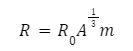R= radius of the nucleus of the element

A= mass number of the element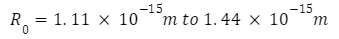## Practice Problems:

Q1. Calculate the total number of protons present in one molecule of NH3.

A. 10
B. 7
C. 13
D. 15

Solution: number of protons in one atom of N (N714) = 7

number of proton in one atom of H (H11) = 1

Total number of protons in 1 molecule of NH3 =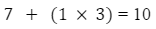Q2. The number of neutrons in dispositive zinc ion with mass number 70 is [IIT 1979]

A. 34
B. 36
C. 38
D. 40

Solution: Zn2+ ioncontains 30 protons and (70 - 30 = 40) neutrons.

Zn3070 contains 30 electrons but Zn2+ iononly 28 electrons.

Q3. Calculate the total number of nucleons present in the Na1123atom.

A. 23
B. 11
C. 22
D. 12

Solution: Atomic number of Na (Z) =11

Mass number of Na = 23

Number of protons per Na atom =11

Number of neutrons per Na atom =23-11=12

Total number of nucleons per atom = 23

Q4. Calculate the total number of neutrons present in one mole of H2O molecules. [NA refers Avogadro's number]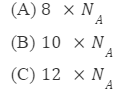D. None of these

Number of neutrons per atom of H(H11)= 1-1=0

Number of neutrons per atom of O(O816)= 16-8=8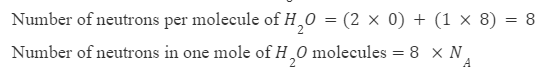Question 1. Can mass numbers be fractions?
Answer: No, the mass number is the sum of the number of protons and neutrons present in the nucleus. It can never be a fraction.

Question 2. what is the difference between mass number and atomic mass?
Answer: generally, Atomic mass is the weighted average mass of an atom of an element based on the relative natural abundance of that element's isotopes. The mass number is the total number of protons and neutrons in an atom's nucleus.

Question 3. What is the unit of mass number?
Answer: mass number is unitless because it is the total number of nucleons present in the atom nucleus but the atomic mass is represented in amu or ‘u’.

Question 4. Why mass of electrons is not considered during the calculation of atomic mass?
Answer: mass of a single electron is approximately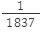times lighter than the mass of the proton. Mass of a neutron is higher than the mass of a proton but for numerical simplicity, we treat the mass of a proton as equal to the mass of the neutron.

Related Topics:

 Electronic configuration Rutherford's atomic model Quantum numbers Isotopes Isobars, Isotones & Isodiaphers Electronic configuration-Afbau Principle, Hund's rule and Pauli's Exclusion principle Thomson's atomic modelTalk to our expert
Resend OTP Timer =
By submitting up, I agree to receive all the Whatsapp communication on my registered number and Aakash terms and conditions and privacy policy